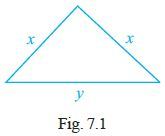#### Write the correct answer.The perimeter of the triangle shown in Fig. 7.1 is(A) 2x + y (B) x + 2y (C) x + y (D) 2x – y

The perimeter of the triangle = sum of the length of the sides

=x+x+y=2x+y

(A) 2x + y